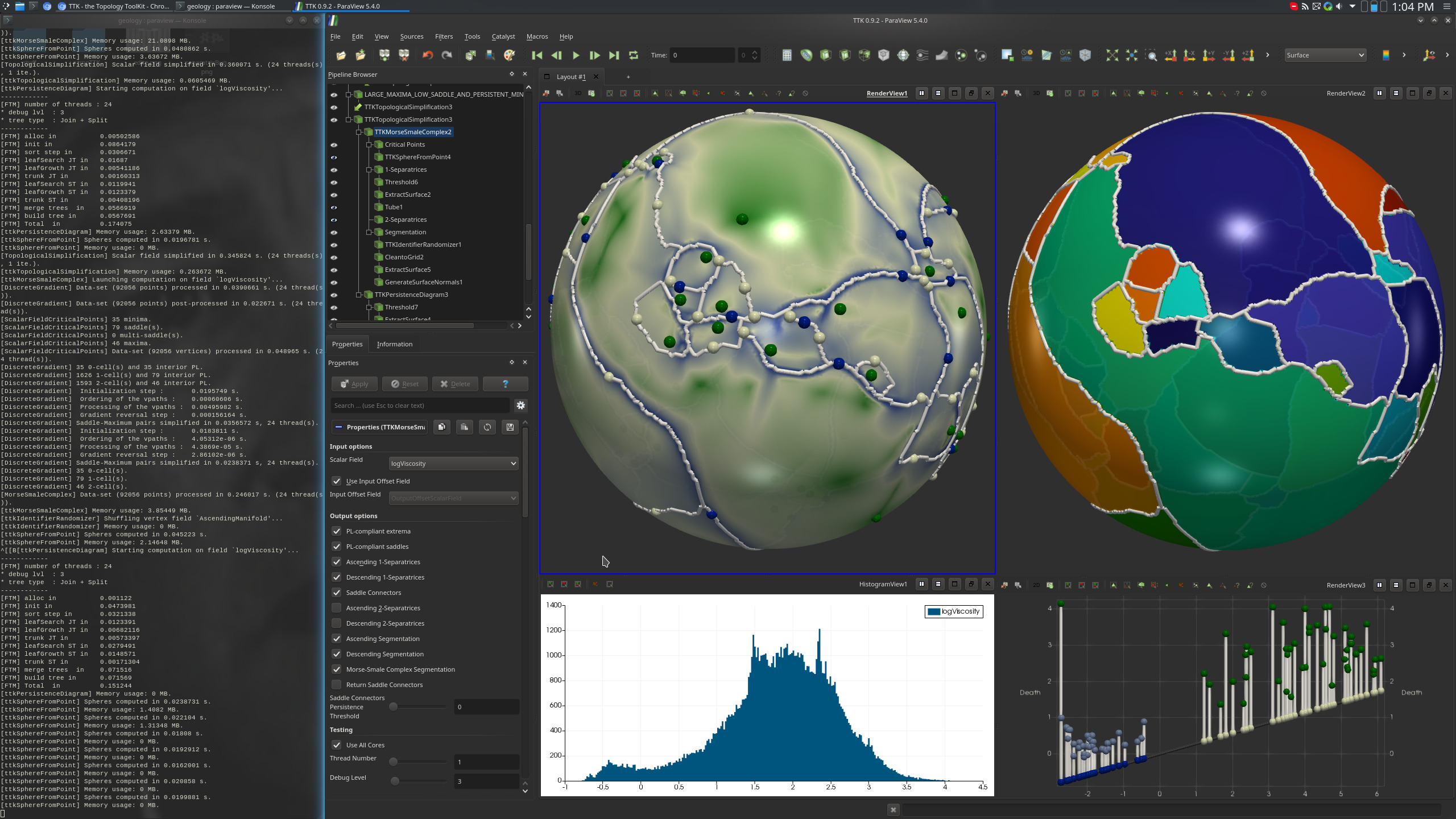# Tectonic Puzzle¶## Pipeline description¶

This example processes a two-dimensional geophysics model of the Earth surface to segment it according to the tectonic plates.

The outer surface of the data-set is first extracted with a combination of ParaView's `Connectivity` and `Threshold`. Then, the `log10` of the `Viscosity` scalar field is computed with a `Calculator` (bottom-right view on the above screenshot).

Several passes of topological simplification are then combined, using PersistenceDiagram, TopologicalSimplification and MorseSmaleComplex to further clean the scalar field. The Persistence Diagram of the scalar field at the end of this cleaning step is represented in the bottom-right view on the above screenshot.

Once this is done, since the low values of the scalar field represent the plates borders and the regions of high values the plates themselves, the `Descending 1-Separatrices` of the Morse-Smale Complex follow the plates borders and the `AscendingManifold` Segmentation of the Morse-Smale Complex gives us the expected segmentation of the tectonic plates (top-right view on the above screenshot).

Finally, the IdentifierRandomizer filter is used to color neighbor cells with a distinct color (top right view on the above screenshot).

## ParaView¶

To reproduce the above screenshot, go to your ttk-data directory and enter the following command:

``````paraview states/tectonicPuzzle.pvsm
``````

## Python code¶

 ``` 1 2 3 4 5 6 7 8 9 10 11 12 13 14 15 16 17 18 19 20 21 22 23 24 25 26 27 28 29 30 31 32 33 34 35 36 37 38 39 40 41 42 43 44 45 46 47 48 49 50 51 52 53 54 55 56 57 58 59 60 61 62 63 64 65 66 67 68 69 70 71 72 73 74 75 76 77 78 79 80 81 82 83 84 85 86 87 88 89 90 91 92 93 94 95 96 97 98 99 100 101 102 103 104 105 106 107 108 109 110 111 112 113 114 115 116 117 118 119 120 121 122 123 124 125 126 127 128 129 130 131 132 133 134 135 136 137 138 139 140 141 142 143 144 145 146 147 148 149 150 151 152 153 154 155 156 157 158 159 160``` ``````#!/usr/bin/env python from paraview.simple import * # create a new 'XML Unstructured Grid Reader' tectonicPuzzlevtu = XMLUnstructuredGridReader(FileName=["tectonicPuzzle.vtu"]) # create a new 'Extract Surface' extractSurface1 = ExtractSurface(Input=tectonicPuzzlevtu) # create a new 'Clean to Grid' cleantoGrid1 = CleantoGrid(Input=extractSurface1) # create a new 'Tetrahedralize' tetrahedralize1 = Tetrahedralize(Input=cleantoGrid1) # create a new 'Connectivity' connectivity1 = Connectivity(Input=tetrahedralize1) # create a new 'Threshold' threshold1 = Threshold(Input=connectivity1) threshold1.Scalars = ["POINTS", "RegionId"] threshold1.ThresholdMethod = "Between" threshold1.LowerThreshold = 1.0 threshold1.UpperThreshold = 1.0 # create a new 'Calculator' calculator1 = Calculator(Input=threshold1) calculator1.ResultArrayName = "logViscosity" calculator1.Function = "log10(Viscosity)" # create a new 'TTK PersistenceDiagram' tTKPersistenceDiagram1 = TTKPersistenceDiagram(Input=calculator1) tTKPersistenceDiagram1.ScalarField = ["POINTS", "logViscosity"] # create a new 'Threshold' threshold2 = Threshold(Input=tTKPersistenceDiagram1) threshold2.Scalars = ["CELLS", "PairIdentifier"] threshold2.ThresholdMethod = "Between" threshold2.LowerThreshold = -0.1 threshold2.UpperThreshold = 999999999 # create a new 'Threshold' persistenceThreshold = Threshold(Input=threshold2) persistenceThreshold.Scalars = ["CELLS", "Persistence"] persistenceThreshold.ThresholdMethod = "Between" persistenceThreshold.LowerThreshold = 0.5 persistenceThreshold.UpperThreshold = 999999999 # create a new 'TTK IcospheresFromPoints' tTKIcospheresFromPoints1 = TTKIcospheresFromPoints(Input=persistenceThreshold) tTKIcospheresFromPoints1.Radius = 0.5 # create a new 'TTK TopologicalSimplification' tTKTopologicalSimplification1 = TTKTopologicalSimplification( Domain=calculator1, Constraints=persistenceThreshold ) tTKTopologicalSimplification1.ScalarField = ["POINTS", "logViscosity"] # create a new 'TTK MorseSmaleComplex' tTKMorseSmaleComplex1 = TTKMorseSmaleComplex(Input=tTKTopologicalSimplification1) tTKMorseSmaleComplex1.ScalarField = ["POINTS", "logViscosity"] # create a new 'TTK IcospheresFromPoints' tTKIcospheresFromPoints2 = TTKIcospheresFromPoints(Input=tTKMorseSmaleComplex1) tTKIcospheresFromPoints2.Radius = 0.1 # create a new 'Threshold' threshold3 = Threshold(Input=tTKIcospheresFromPoints2) threshold3.Scalars = ["POINTS", "CellDimension"] threshold3.ThresholdMethod = "Between" threshold3.LowerThreshold = 2.0 threshold3.UpperThreshold = 2.0 # create a new 'Threshold' lARGE_MAXIMA_THRESHOLD = Threshold(Input=threshold3) lARGE_MAXIMA_THRESHOLD.Scalars = ["POINTS", "ManifoldSize"] lARGE_MAXIMA_THRESHOLD.ThresholdMethod = "Between" lARGE_MAXIMA_THRESHOLD.LowerThreshold = 75.0 lARGE_MAXIMA_THRESHOLD.UpperThreshold = 999999999 # create a new 'Threshold' pERSISTENT_MINIMA = Threshold(Input=tTKIcospheresFromPoints1) pERSISTENT_MINIMA.Scalars = ["POINTS", "CriticalType"] # create a new 'Append Datasets' pERSISTENT_MINIMA_AND_LARGE_MAXIMA = AppendDatasets( Input=[pERSISTENT_MINIMA, lARGE_MAXIMA_THRESHOLD] ) # create a new 'TTK TopologicalSimplification' tTKTopologicalSimplification2 = TTKTopologicalSimplification( Domain=tTKTopologicalSimplification1, Constraints=pERSISTENT_MINIMA_AND_LARGE_MAXIMA ) tTKTopologicalSimplification2.ScalarField = ["POINTS", "logViscosity"] # create a new 'TTK PersistenceDiagram' tTKPersistenceDiagram2 = TTKPersistenceDiagram(Input=tTKTopologicalSimplification2) tTKPersistenceDiagram2.ScalarField = ["POINTS", "logViscosity"] # create a new 'Threshold' threshold4 = Threshold(Input=tTKPersistenceDiagram2) threshold4.Scalars = ["CELLS", "PairIdentifier"] threshold4.ThresholdMethod = "Between" threshold4.LowerThreshold = -0.1 threshold4.UpperThreshold = 999999999 # create a new 'Threshold' threshold5 = Threshold(Input=threshold4) threshold5.Scalars = ["CELLS", "PairType"] threshold5.ThresholdMethod = "Between" threshold5.LowerThreshold = 1.0 threshold5.UpperThreshold = 1.0 # create a new 'Calculator' calculator2 = Calculator(Input=threshold5) calculator2.ResultArrayName = "SaddleValue" calculator2.Function = "coordsX" # create a new 'Threshold' sADDLE_VALUE_THRESHOLD = Threshold(Input=calculator2) sADDLE_VALUE_THRESHOLD.Scalars = ["POINTS", "SaddleValue"] sADDLE_VALUE_THRESHOLD.ThresholdMethod = "Between" sADDLE_VALUE_THRESHOLD.LowerThreshold = -0.2 sADDLE_VALUE_THRESHOLD.UpperThreshold = 1.75 # create a new 'TTK IcospheresFromPoints' tTKIcospheresFromPoints3 = TTKIcospheresFromPoints(Input=sADDLE_VALUE_THRESHOLD) tTKIcospheresFromPoints3.Radius = 0.5 # create a new 'Threshold' lARGE_MAXIMA_LOW_SADDLE = Threshold(Input=tTKIcospheresFromPoints3) lARGE_MAXIMA_LOW_SADDLE.Scalars = ["POINTS", "CriticalType"] lARGE_MAXIMA_LOW_SADDLE.ThresholdMethod = "Between" lARGE_MAXIMA_LOW_SADDLE.LowerThreshold = 3.0 lARGE_MAXIMA_LOW_SADDLE.UpperThreshold = 3.0 # create a new 'Append Datasets' lARGE_MAXIMA_LOW_SADDLE_AND_PERSISTENT_MINIMA = AppendDatasets( Input=[pERSISTENT_MINIMA, lARGE_MAXIMA_LOW_SADDLE] ) # create a new 'TTK TopologicalSimplification' tTKTopologicalSimplification3 = TTKTopologicalSimplification( Domain=tTKTopologicalSimplification2, Constraints=lARGE_MAXIMA_LOW_SADDLE_AND_PERSISTENT_MINIMA, ) tTKTopologicalSimplification3.ScalarField = ["POINTS", "logViscosity"] # create a new 'TTK MorseSmaleComplex' tTKMorseSmaleComplex2 = TTKMorseSmaleComplex(Input=tTKTopologicalSimplification3) tTKMorseSmaleComplex2.ScalarField = ["POINTS", "logViscosity"] # create a new 'TTK IdentifierRandomizer' tTKIdentifierRandomizer1 = TTKIdentifierRandomizer( Input=OutputPort(tTKMorseSmaleComplex2, 3) ) tTKIdentifierRandomizer1.ScalarField = ["POINTS", "AscendingManifold"] SaveData("Segmentation.vtu", tTKIdentifierRandomizer1) ``````

To run the above Python script, go to your ttk-data directory and enter the following command:

``````pvpython python/tectonicPuzzle.py
``````

## Inputs¶

• tectonicPuzzle.vtu: two 2-dimensional spheres (one around the other) made up of a triangular mesh. There are several point data arrays attached to it. Those scalar fields represent geophysics measures on the earth surface (and at a certain depth under it); only the `Viscosity` field will be used in the current example.

## Outputs¶

• `Segmentation.vtu`: the output segmentation in VTK file format (top right view, above screenshot). This corresponds to a segmentation of the tectonic plates from the `Viscosity` scalar field.

## C++/Python API¶

IcospheresFromPoints

IdentifierRandomizer

MorseSmaleComplex

PersistenceDiagram

TopologicalSimplification